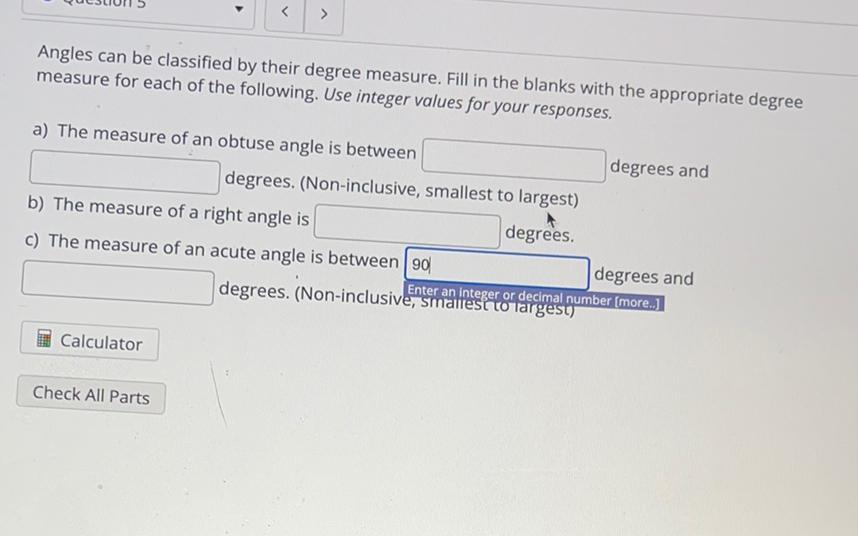Question:

# > Angles can be classified by their degree measure. Fill in the blanks with the appropriate degree measure for each of the follo> Angles can be classified by their degree measure. Fill in the blanks with the appropriate degree measure for each of the following. Use integer values for your responses. a) The measure of an obtuse angle is between degrees and degrees. (Non-inclusive, smallest to largest) b) The measure of a right angle is degrees. c) The measure of an acute angle is between 90 degrees and Enter an integer or decimal number (more.] degrees. (Non-inclusive, smallest to largest) Calculator Check All Parts